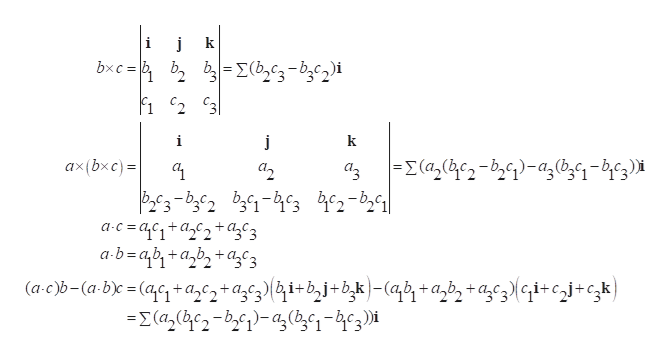For any vectors a, b, and c, prove thata X (b X c) = (a*c)b - (a*b)c

Question

For any vectors a, b, and c, prove that

a X (b X c) = (a*c)b - (a*b)c

Step 1

Calculation:

Let us assume that

Step 2help_outlineImage Transcriptionclosej k bxc= b2 b-Σ-b,c,i C3 i j ΕΣ((-b)-a,6,9- b3) x(bxc) = 2 ac=c1+a2+3 ab=ab+azb2+3 (a-c)b-(a-b)c =(ac1+acz+agcz}{&i+bj+bk)-(qb1 + a_b» +azc3}Gi+cj+cgk} -X(a2 c2-b)-a,b,-4¢3)i fullscreen

Want to see the full answer?

See Solution

Want to see this answer and more?

Our solutions are written by experts, many with advanced degrees, and available 24/7

See Solution
Tagged in

Other